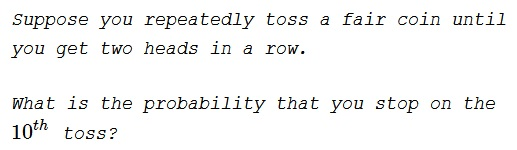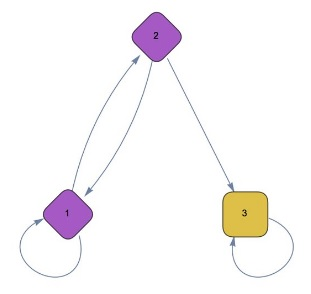# Two in a Row

### Problem### Solution 1

Let $X_n$ stand for the result of $n^{th}$ toss. We'll look into a more general problem of having to stop at the $n^{th}$ toss. This would mean $X_n=X_{n-1}=H$ and $X_{n-2}=T.$ In addition, the sequence of the previous tosses should not contain two consecutive heads.

Such a sequence may start with either $T$ or $HT$ and the remainder ought to be a sequence with the same property - no $HH.$ Thus we get a recurrence $S_k=S_{k-1}+S_{k-2}.$ Where $S_k$ is the number of sequence with no $HH$ of length $k.$ Obviously, $S_1=2,$ $S_2=3,$ meaning $S_k=F_{k+2},$ where $F_n$ is the Fibonacci sequence

$0,1,1,2,3,5,8,13,21,34,\cdots$ and $0=F_0.$

There are $2^n$ possible outcomes for $n$ tosses of a coin; of these $S_{n-3}$ end with $THH$ and have no repeated heads beforehand. So the sought probability is $\displaystyle P_n=\frac{F_{n-1}}{2^n},$ which for $n=10$ gives $\displaystyle P_{10}=\frac{34}{1024}.$

### Solution 2

Let $Q_k$ be the event that two consecutive heads do not occur in $k$ consecutive tosses.

A $Q_k$ event ends in one of the following patterns: $TH$, $TT$, and $HT$. Suppose, the coin is tossed one more time. The probability of $Q_{k+1}$ would be the same as $Q_k$ if not for a possible ending pattern of $THH$ arising from the $TH$ ending pattern in $Q_k$. Thus, we need to subtract of the probability of the $THH$ ending. Hence,

$\displaystyle P(Q_{k+1})=P(Q_k)-\frac{1}{8}P(Q_{k-2}).$

Solving for this recurrence relation,

$\displaystyle P(Q_k)=c_1\left(\frac{1}{2}\right)^k+c_2\left[\frac{(1-\sqrt{5})}{4}\right]^k+c_3\left[\frac{(1+\sqrt{5})}{4}\right]^k.$

$c_1$, $c_2$, and $c_3$ are undetermined coefficients that can be solved for using the initial conditions: $P(Q_1)=1$, $P(Q_2)=3/4$, and $P(Q_3)=5/8$ (obtained by enumerating the cases). This results in

$\displaystyle P(Q_k)=\frac{(5-3\sqrt{5})}{10}\left[\frac{(1-\sqrt{5})}{4}\right]^k+\frac{(5+3\sqrt{5})}{10}\left[\frac{(1+\sqrt{5})}{4}\right]^k.$

For ending with two consecutive heads for the first time on the $10^{th}$ toss, $Q_7$ has to happen followed by $THH$. Thus, the required probability is

$\displaystyle \frac{1}{8}P(Q_7)=\frac{(5-3\sqrt{5})}{80}\left[\frac{(1-\sqrt{5})}{4}\right]^7+\frac{(5+3\sqrt{5})}{80}\left[\frac{(1+\sqrt{5})}{4}\right]^7=\frac{17}{512}.$

### Solution 3

We have the following Markov Chain:with the following transition matrix $M$:

$\begin{array}{cccc} & \text{N} & \text{H} & \text{HH} \\ \text{N} & \frac{1}{2} & \frac{1}{2} & 0 \\ \text{H} & \frac{1}{2} & 0 & \frac{1}{2} \\ \text{HH} & 0 & 0 & 1 \\ \end{array}$

The matrix $M$ to the $i^{th}$ power:

$\small{ \left( \begin{array}{ccc} \frac{2^{-2 i-1} \left(\sqrt{5}-1\right) \left(1-\sqrt{5}\right)^i}{\sqrt{5}}+\frac{2^{-2 i-1} \left(\sqrt{5}+1\right)^{i+1}}{\sqrt{5}} & \frac{2^{-2 i-2} \left(\sqrt{5}-1\right) \left(\sqrt{5}+1\right)^{i+1}}{\sqrt{5}}-\frac{2^{-2 i-2} \left(1-\sqrt{5}\right)^i \left(\sqrt{5}-1\right) \left(\sqrt{5}+1\right)}{\sqrt{5}} & \frac{2^{-2 i-2} \left(\sqrt{5}-1\right)^2 \left(1-\sqrt{5}\right)^i}{\sqrt{5}}-\frac{2^{-2 i-2} \left(\sqrt{5}+1\right)^{i+2}}{\sqrt{5}}+1 \\ \frac{\left(\frac{1}{4} \left(\sqrt{5}+1\right)\right)^i}{\sqrt{5}}-\frac{\left(\frac{1}{4} \left(1-\sqrt{5}\right)\right)^i}{\sqrt{5}} & \frac{2^{-2 i-1} \left(\sqrt{5}+1\right) \left(1-\sqrt{5}\right)^i}{\sqrt{5}}+\frac{2^{-2 i-1} \left(\sqrt{5}-1\right) \left(\sqrt{5}+1\right)^i}{\sqrt{5}} & -\frac{2^{-2 i-1} \left(\sqrt{5}-1\right) \left(1-\sqrt{5}\right)^i}{\sqrt{5}}-\frac{2^{-2 i-1} \left(\sqrt{5}+1\right)^{i+1}}{\sqrt{5}}+1 \\ 0 & 0 & 1 \\ \end{array} \right) }$

The matrix $M$ after $i$ steps starting from position 1:

$\displaystyle \left(\begin{array}\,1\\0\\0\end{array}\right)^T\cdot\text{M}^i=\left( \begin{array}{c} \displaystyle \frac{2^{-2 i-1} \left(\left(1+\sqrt{5}\right)^{i+1}-\left(1-\sqrt{5}\right)^{i+1}\right)}{\sqrt{5}} \\ \displaystyle \frac{4^{-i} \left(\left(1+\sqrt{5}\right)^i-\left(1-\sqrt{5}\right)^i\right)}{\sqrt{5}} \\ \displaystyle \frac{1}{5} 2^{-2 i-1} \left(5\cdot 2^{2 i+1}+\left(3 \sqrt{5}-5\right) \left(1-\sqrt{5}\right)^i-\left(5+3 \sqrt{5}\right) \left(1+\sqrt{5}\right)^i\right) \\ \end{array} \right)$

Hence the cumulative probability $P$ of having HH in steps $i$ or less:

$P(i) =\frac{1}{5} 2^{-2 i-1} \left(\left(3 \sqrt{5}-5\right) \left(1-\sqrt{5}\right)^i+5\ 2^{2 i+1}-\left(3 \sqrt{5}+5\right) \left(\sqrt{5}+1\right)^i\right)$

which yields the mass generating function:

$p(i)=P(i)-P(i-1)= \frac{1}{5} 2^{-2 i-1} \left(\left(\sqrt{5}+5\right) \left(1-\sqrt{5}\right)^i-\left(\sqrt{5}-5\right) \left(\sqrt{5}+1\right)^i\right)$

By the Binet identity, where $F$ is the Fibonacci number,

$F_{i-1}=\frac{\left(\sqrt{5}+5\right)^{i-1}-\left(\sqrt{5}-5\right)^{i-1}}{2^{i-1} \sqrt{5}}\\ p(i)=\frac{F_{i-1}}{2^i}$

here

$p(10)=\frac{34}{1024}$.

Unconditional probability of being in $2^{nd}$ game (conditional on both winning previous games but regardless of having been matched): $\displaystyle p_2=(\frac{1}{2^2}) \frac{n/2^2}{n/2 \choose 2}$

Unconditional probability of being in $i^{th}$ game: $\displaystyle p_i=(\frac{1}{2^i}) \frac{\frac{n}{2^i}}{n/2^{i-1} \choose 2}$

Conditional probability of being in $i^{th}$ game: $p'_i=(1-p_{i-1})(\frac{1}{2^i}) \frac{\frac{n}{2^i}}{n/2^{i} \choose 2}$

$p_1 +(1-p_1) p_2+ (1-p_1)(1-p_2) p_3$

$\displaystyle P(n)=\sum _{k=1}^m p_k \prod _{j=1}^{k-1} (1-p_j), \;; m=\frac{\log{n}}{\log{2}}$

### Solution 4### Solution 5

For $10$ tosses, the $9^{th}$ and the $10^{th}$ should be heads $(H).$ So we concentrated on the first eight tosses:

1. All tails, $\displaystyle {8\choose 0}=1.$
2. One head, $7$ tails. There are $7$ possible positions of $H$ before and after $T,$ excluding the very last - after all tails. ${7\choose 1}=7.$
3. $2H,$ $6T.$ Same reasoning as above, $6$ distinct position for $H$ between $T's$, including upfront: ${6\choose 2}=15.$
4. and so on

The total probability is the sum of the cases above, divided by $2^{10}:$

$\displaystyle P(10)=\frac{8\choose 0}+{7\choose 1}+{6\choose 2}+{5\choose 3}+{4\choose 4}}{2^{10}}=\frac{34}{1024}$

In general, the formula is

$\displaystyle P(n)=\frac{1}{2^n}\sum_{i=0}^{(n-2)/2}{n-2-i\choose i}.$

### Acknowledgment

This is problem P1983-2 from A Friendly Mathematics Competition by Rick Gillman (MAA, 2003)

Solution 2 is by Amit Itagi; Solution 3 is by N. N. Taleb; Solution 4 is by Attila Kun; Solution 5 is by Giorgos Papadopulos.